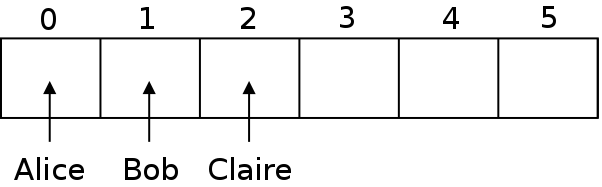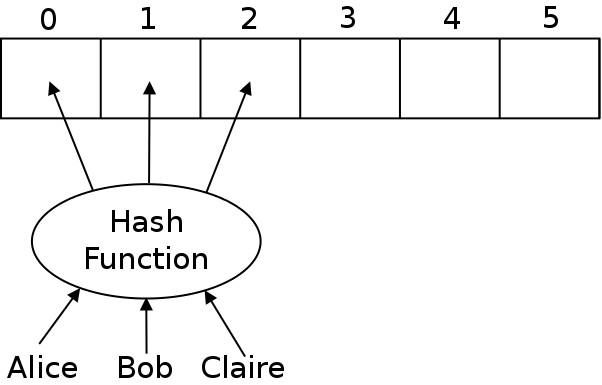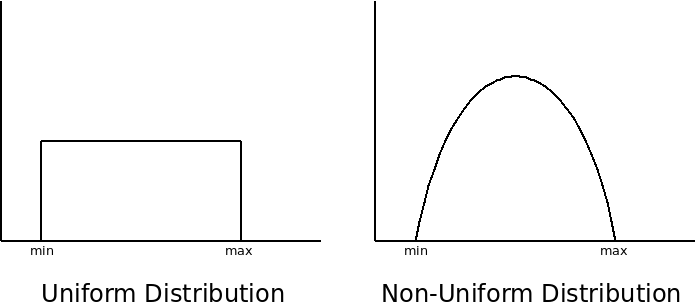# Hash Tables

### Overview

Efficiently searchable data is important for many applications. We have seen a few data structures capable of searching:

 Data Structure Searching Insertion Unsorted Array/Linked List Sorted Array Binary Search Tree

The absolute best case scenario for this would be if searching and insertion were $O(1)$.

This is the goal of hash tables.

### Hash Tables

The idea behind a hash table is that we make an array of our items. Then we put each item into an array slot where we will know where to get it when we need it. For example, if we have the people "Alice", "Bob", and "Claire", then we can put "Alice" in slot 0, "Bob" in slot 1 and "Claire" in slot 2. Then if we need to look up one of their numbers, we can just look in the same place we stored them.

A hash table has keys and values. The keys are whatever we will use to search. In a phonebook program, the keys will be names.

The values are the actual data we want to store inside of the hash table. In a phonebook program, this would be the phone numbers and addresses.However, the problem is how can we decide what slot to put our data in?

### Hash Functions

The way that we can decide where to put each entry is by using a hash function. A hash function takes our keys that we want to store, and picks a number for it. That number will be used as an index into the array.The hash function will map the keys to integers. It must take any key and return the integer to store it at.

The hash function must always return the same number for the same object. That way, when we store the object, we will put it into a given location. Then, when we are searching for it, all we will need to do is run the hash function which will tell us which slot to look in.

If the keys are integers, then the problem is fairly easy. We can just use the keys directly as indices into the table.

However, one issue we have to contend with is making sure that the indices we use are never out of range of the table. For example, if we are using social security numbers as our keys, then the keys will be 9-digit numbers. To use a 9-digit index, the table would need to be one billion entries big.

To avoid this, we can just modulus by the size of the table:


int hash(int key) {
return key % TABLE_SIZE;
}


But what about using strings as a key? There are many strategies we can use. A simple one is to add up the ASCII values of each character in the string. Then we can take that total and then again modulus by the size of the table:

How can we implement this in C++?





### Collision Resolution

The example above has an issue, which is that it doesn't handle collisions. A collision occurs when the hash function returns the same index for two different keys. Because the possible number of keys is typically much larger than the number of valid indices, collisions are always possible.

One way of handling collisions is to check if the place we want to store an element is occupied. If it is, we move onto the next element, repeatedly until we find an empty slot to insert our data. This is called linear probing since we go in a line looking for an empty slot.

How could we add this to our hash table?





### Chained Hash Tables

Another approach to solving collisions, is called "chaining". What we do here is keep the hash table as an array of linked lists. Each time we want to insert an item into the hash table, we will hash the key, which will tell us which slot in the array to use. Then the item will be appended to the given linked list.What are the trade-offs of linear probing vs. chaining?





### Performance Analysis

The goal of hash tables was to make searching a $O(1)$ operation. The lookup code when using a chained hash tabel might look like this:


void lookup(const char* name) {
int index = hash(name);

Node* current = phonebook[index];
int number = 0;
while(strcmp(current->person.name, name) != 0) {
number++;
current = current->next;
}

return phonebook[index].number;
}


The hash function only looks at the name itself, it doesn't matter how many things are in the table, so that function will be $O(1)$. Then we have the loop that goes through the chain until it finds what we are looking for. Just looking at this, it looks like it would be $O(N)$ because of the while loop.

In the worst case, the hash function always returns the same index, and all of our items are stored in the same list. When we need to search for an item, then, we will just be searching though a linked list (or an array if we are using linear probing) for our item. This would be $O(n)$.

In the best case, however, we will have a large enough table to fit all of our data easily, and the hash function will return a different index for each key. In this case, each item will be exactly where the hash says it will be, there will be no looping needed, and the search is $O(1)$.

When using hash tables, the table should be significantly larger than the most data you will have. Also the hash function should provide a uniform distribution of indices:With a large table, and a well-designed hash function, there will be minimal collisions, so searching a hash table is $O(1)$ in general.

Another method for resolving collisions is called rehashing. With this technique, we would have a second hash function that is used if the first one causes a collision. If the second one also causes a collision, then we have to resort to linear probing or chaining. Rehashing avoids problems where our input function has a "spike" in one particular area of the table. Running them through a second hash function can help smooth this out.

### Conclusion

Hash tables require more tweaking than most other data structures. The size of the table must be carefully chosen. If the table fills up too much, it slows down. Also, unlike most data structures, hash tables cannot be easily resized since the hash function would need to return new results.

The hash function also must be carefully chosen. If it does a poor job, performance will suffer.

If all of the data is known ahead of time, one can write a perfect hash function that assigns a different index to each piece of data. Generally though, the hash function doesn't know what data will be used ahead of time, and must try to use as uniform a distribution as possible.

If the hash table is well-tuned, it is the fastest way to store searchable data.